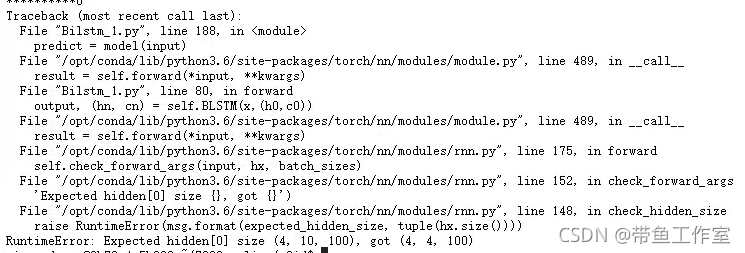# RuntimeError: Expected hidden size (x, x, x), got(x, x, x)The above figure shows the problem when training the bilstm network.

Problem Description: define the initial weights H0 and C0 of bilstm network and input them to the network as the initial weight of bilstm, which is realized by the following code

``output, (hn, cn) = self.bilstm(input, (h0, c0))``

The network structure is as follows:

``````self.bilstm = nn.LSTM(
input_size=self.input_size,
hidden_size=self.hidden_size,
num_layers=self.num_layers,
bidirectional=True,
bias=True,
dropout=config.drop_out
)``````

The dimension of initial weight is defined as   H0 and C0 are initialized. The dimension is:

``````**h_0** of shape `(num_layers * num_directions, batch, hidden_size)`
**c_0** of shape `(num_layers * num_directions, batch, hidden_size)```````

In bilstm network, the parameters are defined as follows:

``````num_layers: 2

num_directions: 2

batch: 4

seq_len: 10

input_size: 300

hidden_size: 100 ``````

Then according to the definition in the official documents    H0, C0 dimensions should be: (2 * 2, 4100) = (4, 4100)

However, according to the error screenshot at the beginning of the article, the dimension of the initial weight of the hidden layer should be (4, 10100), which makes me doubt whether the dimension specified in the official document is correct.

Obviously, the official documents cannot be wrong, and the hidden state dimensions when using blstm, RNN and bigru in the past are the same as those specified by the official, so I don’t know where to start.

Therefore, we re examined the network structure and found that an important parameter, batch, was missing_ First, let’s take a look at all the parameters required by bilstm:

``````Args:
input_size: The number of expected features in the input `x`
hidden_size: The number of features in the hidden state `h`
num_layers: Number of recurrent layers. E.g., setting ``num_layers=2``
would mean stacking two LSTMs together to form a `stacked LSTM`,
with the second LSTM taking in outputs of the first LSTM and
computing the final results. Default: 1
bias: If ``False``, then the layer does not use bias weights `b_ih` and `b_hh`.
Default: ``True``
batch_first: If ``True``, then the input and output tensors are provided
as (batch, seq, feature). Default: ``False``
dropout: If non-zero, introduces a `Dropout` layer on the outputs of each
LSTM layer except the last layer, with dropout probability equal to
:attr:`dropout`. Default: 0
bidirectional: If ``True``, becomes a bidirectional LSTM. Default: ``False````````

batch_ The first parameter can make the dimension batch in the first dimension during training, that is, the input data dimension is

(batch size, SEQ len, embedding dim), if not added   batch_ First = true, the dimension is

（seq len，batch size，embedding dim）

Because there was no break at noon, I vaguely forgot to add this important parameter, resulting in an error: the initial weight dimension is incorrect, and I can add it   batch_ Run smoothly after first = true.

The modified network structure is as follows:

``````self.bilstm = nn.LSTM(
input_size=self.input_size,
hidden_size=self.hidden_size,
num_layers=self.num_layers,
batch_first=True,
bidirectional=True,
bias=True,
dropout=config.drop_out
)``````

Extension: when we use RNN and its variant network, if we want to add the initial weight, the dimension must be the officially specified dimension, i.e

`(num_layers * num_directions, batch, hidden_size)`

At the same time, be sure to set batch_ First = true. The official document does not specify when batch is set_ When first = true, the dimensions of H0, C0, HN and CN are (num_layers * num_directions, batch, hidden_size), so be careful!

At the same time, check whether batch is set when the dimensions of HN and CN are incorrect_ First parameter, RNN and its variant networks are applicable to this method!